# Package Modelica.​Math.​SpecialLibrary of special mathematical functions

### Information

This sublibrary contains functions to compute often used mathematical operators that cannot be expressed analytically.

Extends from `Modelica.​Icons.​Package` (Icon for standard packages).

### Package Contents

NameDescription
`erf`Error function erf(u) = 2/sqrt(pi)*Integral_0_u exp(-t^2)*d
`erfc`Complementary error function erfc(u) = 1 - erf(u)
`erfcInv`Inverse complementary error function: u = erfc(erfcInv(u))
`erfInv`Inverse error function: u = erf(erfInv(u))
`Internal`Internal utility functions that should not be directly utilized by the user
`sinc`Unnormalized sinc function: sinc(u) = sin(u)/u

## Function Modelica.​Math.​Special.​erfError function erf(u) = 2/sqrt(pi)*Integral_0_u exp(-t^2)*d

### Information

#### Syntax

```Special.erf(u);
```

#### Description

This function computes the error function erf(u) = 2/sqrt(pi)*Integral_0_u exp(-t^2)*dt numerically with a relative precision of about 1e-15. The implementation utilizes the formulation of the Boost library (53-bit implementation of erf.hpp, developed by John Maddock). Plot of the function: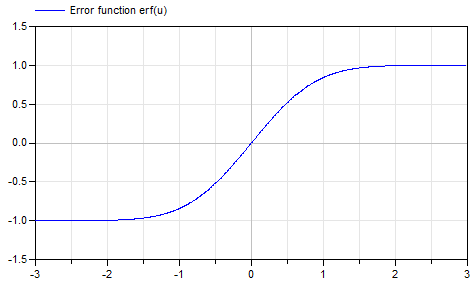For more details, see Wikipedia.

#### Example

```  erf(0)    // = 0
erf(10)   // = 1
erf(0.5)  // = 0.520499877813047
```

Extends from `Modelica.​Icons.​Function` (Icon for functions).

### Inputs

TypeNameDescription
`Real``u`Input argument

### Outputs

TypeNameDescription
`Real``y`= 2/sqrt(pi)*Integral_0_u exp(-t^2)*dt

## Function Modelica.​Math.​Special.​erfcComplementary error function erfc(u) = 1 - erf(u)

### Information

#### Syntax

```Special.erfc(u);
```

#### Description

This function computes the complementary error function erfc(u) = 1 - erf(u) with a relative precision of about 1e-15. The implementation utilizes the formulation of the Boost library (53-bit implementation of erf.hpp developed by John Maddock). Plot of the function: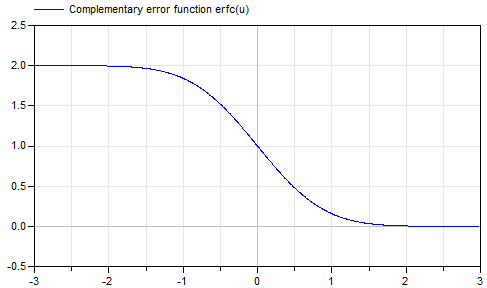If u is large and erf(u) is subtracted from 1.0, the result is not accurate. It is then better to use erfc(u). For more details, see Wikipedia.

#### Example

```  erfc(0)    // = 1
erfc(10)   // = 0
erfc(0.5)  // = 0.4795001221869534
```

Extends from `Modelica.​Icons.​Function` (Icon for functions).

### Inputs

TypeNameDescription
`Real``u`Input argument

### Outputs

TypeNameDescription
`Real``y`= 1 - erf(u)

## Function Modelica.​Math.​Special.​erfInvInverse error function: u = erf(erfInv(u))

### Information

#### Syntax

```Special.erfInv(u);
```

#### Description

This function computes the inverse of the error function erf(u) = 2/sqrt(pi)*Integral_0_u exp(-t^2)*dt numerically with a relative precision of about 1e-15. Therefore, u = erf(erfInv(u)). Input argument u must be in the range (otherwise an assertion is raised):

-1 ≤ u ≤ 1

If u = 1, the function returns Modelica.Constants.inf.
If u = -1, the function returns -Modelica.Constants.inf
The implementation utilizes the formulation of the Boost library (erf_inv.hpp, developed by John Maddock).
Plot of the function: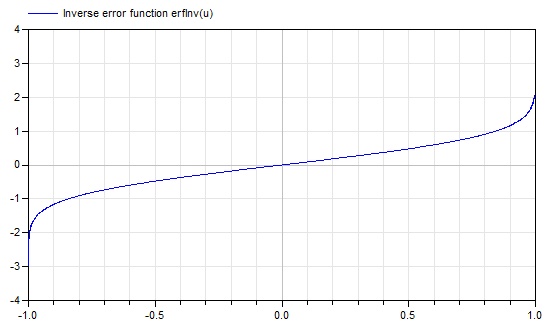For more details, see Wikipedia.

#### Example

```  erfInv(0)            // = 0
erfInv(0.5)          // = 0.4769362762044699
erfInv(0.999999)     // = 3.458910737275499
erfInv(0.9999999999) // = 4.572824958544925
```

Extends from `Modelica.​Icons.​Function` (Icon for functions).

### Inputs

TypeNameDescription
`Real``u`Input argument in the range -1 <= u <= 1

### Outputs

TypeNameDescription
`Real``y`= inverse of error function

## Function Modelica.​Math.​Special.​erfcInvInverse complementary error function: u = erfc(erfcInv(u))

### Information

#### Syntax

```Special.erfInv(u);
```

#### Description

This function computes the inverse of the complementary error function erfc(u) = 1 - erf(u) with a relative precision of about 1e-15. Therefore, u = erfc(erfcInv(u)) and erfcInv(u) = erfInv(1 - u). Input argument u must be in the range (otherwise an assertion is raised):

0 ≤ u ≤ 2

If u = 2, the function returns -Modelica.Constants.inf.
If u = 0, the function returns Modelica.Constants.inf
The implementation utilizes the formulation of the Boost library (erf_inv.hpp, developed by John Maddock).
Plot of the function: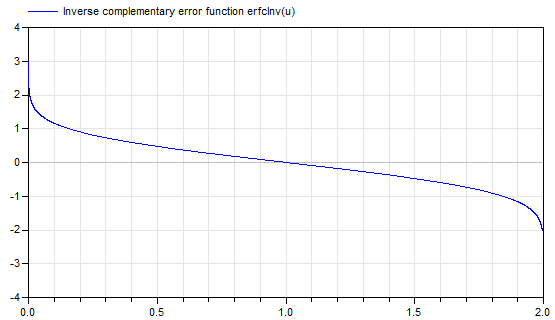For more details, see Wikipedia.

#### Example

```  erfcInv(1)         // = 0
erfcInv(0.5)       // = 0.4769362762044699
erfInv(1.999999)   // = -3.4589107372909473
```

Extends from `Modelica.​Icons.​Function` (Icon for functions).

### Inputs

TypeNameDescription
`Real``u`Input argument

### Outputs

TypeNameDescription
`Real``y`erfcInv(u)

## Function Modelica.​Math.​Special.​sincUnnormalized sinc function: sinc(u) = sin(u)/u

### Information

#### Syntax

```Special.sinc(u);
```

#### Description

This function computes the unnormalized sinc function sinc(u) = sin(u)/u. The implementation utilizes a Taylor series approximation for small values of u. Plot of the function: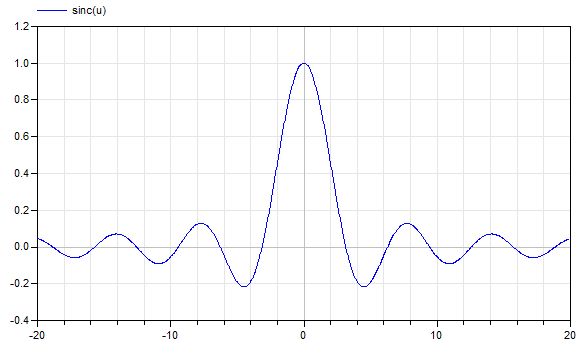For more details, see Wikipedia.

#### Example

```  sinc(0)   // = 1
sinc(3)   // = 0.0470400026866224
```

Extends from `Modelica.​Icons.​Function` (Icon for functions).

### Inputs

TypeNameDescription
`Real``u`Input argument

### Outputs

TypeNameDescription
`Real``y`= sinc(u) = sin(u)/u

Generated 2018-12-12 12:14:35 EST by MapleSim.